## Reduction of Order for Linear 2nd-Order ODE

The Reduction of Order technique is a method for determining a second
linearly independent solution to a homogeneous second-order linear ode
given a first solution. This section has the following:

Example 1

It is best to describe the procedure with a concrete example. Consider the
linear ode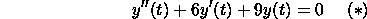We know that a solution to this problem is y_1=exp(-3t). To obtain the
general solution we need a second linearly independent solution to the
problem.

We find the second solution by assuming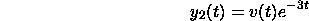where v(t) is an unknown function. We now substitute this into the original
ode (*) and derive a new ode for v(t). We have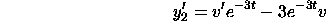and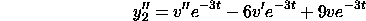Substituting these expressions into (*), we obtain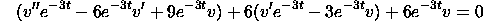Many terms cancel in the above expression. We obtain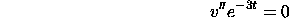Either v''=0 or exp(-3t)=0. The second possibility only occurs if t=infinity
and this is a degenerate case. We must have v''=0. In words: the second
derivative of v is 0. This means that v is a line: v=At+B, where A and B
are constants. Hence, a second solution to the original ode (*) is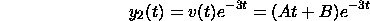How do we choose A and B? Recall, our goal is determine a second linearly
independent solution to the original ode (*). The first solution is y_1=exp(-3t).
Suppose we set A=0. Then y_2=Bexp(-3t). In this case, y_1 and y_2 are
multiples of each other, and are linearly dependent. On the other hand,
suppose we choose B=0. Then y_2=Atexp(-3t). In this case y_1=exp(-3t)
and y_2=Atexp(-3t) are indeed linearly independent. As long as A is
nonzero, y_1 and y_2 are linearly independent. It makes sense to set B=0,
since if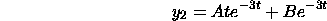the term Bexp-3t is the same as y_1=exp(-3t) and adds nothing new.

To make a long story short(!), we choose A=1 and B=0. The second linearly
independent solution to (*) is y_2=texp(-3t) and the general solution to (*) is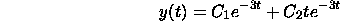General Solution Procedure

Let us now consider a general homogeneous linear ode: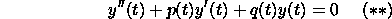and suppose f(t), which is known, is one solution of the ode. That means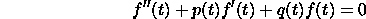We assume the second solution has the form: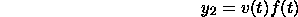where v(t) is unknown. We now substitute y_2 into the original ode (**).
We have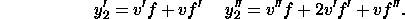Substituting these expressions into (**), we have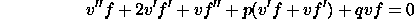Rearranging terms, we have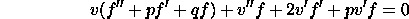The term in parentheses is 0, since f(t) is a solution to (**). Hence, we
are left with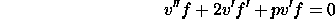Dividing by f, we have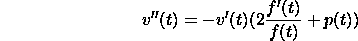This is a linear second-order ode where v'' depends on v' and t only and
can be solved. It can be shown that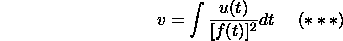where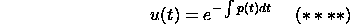It follows that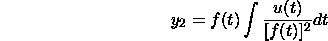#### Example 2

Consider the ode: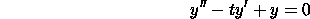It can be shown that y_1(t)=f(t)=t is a solution. We can apply formulas
(****) and (***) to find the second solution. Here p(t)=-t. We have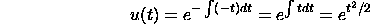Substituting this into (***), we have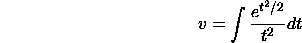This integral cannot be expressed in terms of elementary functions. The
second linearly independent solution is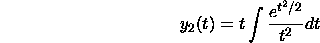The general solution to the ode is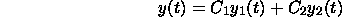Copyright © 1996 Department of Mathematics, Oregon State University

If you have questions or comments, don't hestitate to contact us.